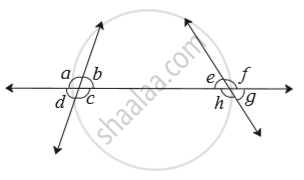# Observe the Angles Shown in the Figure and Write the Following Pair of Angle. Interior Angles - Mathematics

Sum

Observe the angles shown in the figure and write the following pair of angle.Interior angles

#### Solution

Interior angles : A pair of angles which are on the same side of transversal and inside the given lines that are intersected by the transversal.

Interior angles
(a) ∠c and ∠h
(b) ∠b and ∠e

Concept: Pairs of Lines - Transversal
Is there an error in this question or solution?

#### APPEARS IN

Balbharati Mathematics 8th Standard Maharashtra State Board
Chapter 2 Parallel lines and transversals
Practice Set 2.1 | Q 1.3 | Page 8